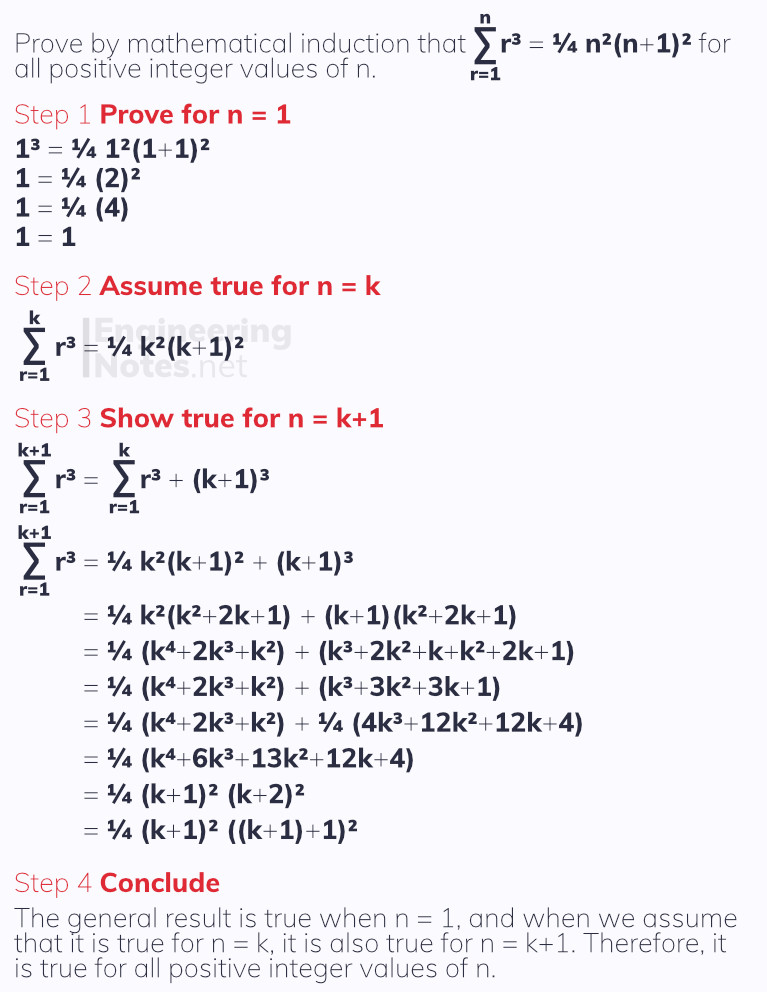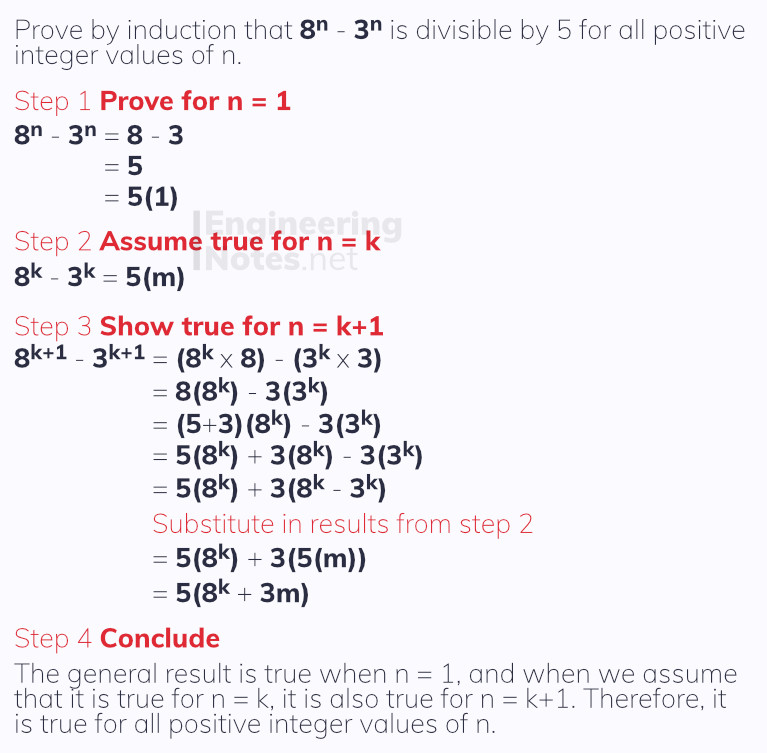#### Notes by Category University Engineering

Electronics*
Mathematics*
Mechanics & Stress Analysis*
Rate these notesNot a fanNot so goodGoodVery goodBrillRate these notes
• A-Level Further Maths

# Proof by Induction

Proof by induction is used to prove that a general statement is true for all positive integer values. All proofs by mathematical induction follow four basic steps:

1. Prove that the general statement is true when n = 1

2. Assume the general statement is true for n = k

3. Show that, if it is true for n = 1, the general statement is also true for n = k+1

4. Conclude that the general statement is true whenever n

All four steps must be shown clearly in your workings: prove, assume, show, conclude.

## Proving Sums

Often, questions will use the standard results for the sums of r, r² and r³. Regardless, the method for all sums is the same and follows the four steps above.### Quick Tip

For the 3rd step, it is generally best to write the last line out first using the general function - just substitute (k+1) into it. You know that this is the answer you want to reach, so use it as a target to help you.

## Proving Divisibility Results

Again, follow the four standard steps for proof by induction. For divisibility results, make step 2 equal any general multiple of the divisor:## Proof Using Matrices

Exactly the same four steps apply: